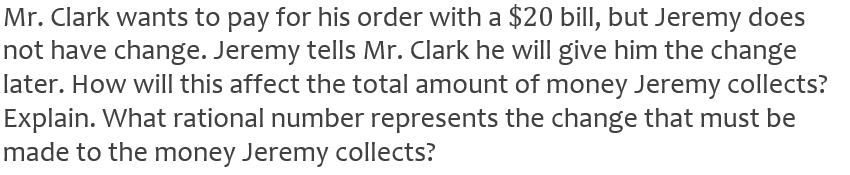Daily Math Challenge October 29, 2018
starstarstarstarstarstarstarstarstarstar
by Beth Mathis
| 2 Questions
Note from the author:
7.NS.A.1 and 7.NS.A.21
1
You are only looking at Aaron's work! There is no other work!
Is Aaron correct or incorrect, why?
Yes, Aaron is correct because there three whole numbers between 5 and 1. The addional distance was added on by adding the fractions together.
Yes, Aaron is correct because to find the distance between two numbers you can subtract and if you subtract the two numbers you do get 3 + 7/12
No, Aaron is incorrect. There are three whole numbers between the two numbers, 1/4 is past the 5. That would increase the distance from 5. However 1/3 is addional 2/3 past the two not 1/3. So he should have added 2/3 and 1/4 to get 11/12. The distance is 3 + 11/12.
No, Aaron is incorrect. To find distance you need to subtract the two numbers. If you subtract the two numbers you get 4 and 1/12.2
1
What rational number represents the change that must be make to the money Jeremy collects.
11.25
-11.25
8.75
-8.75